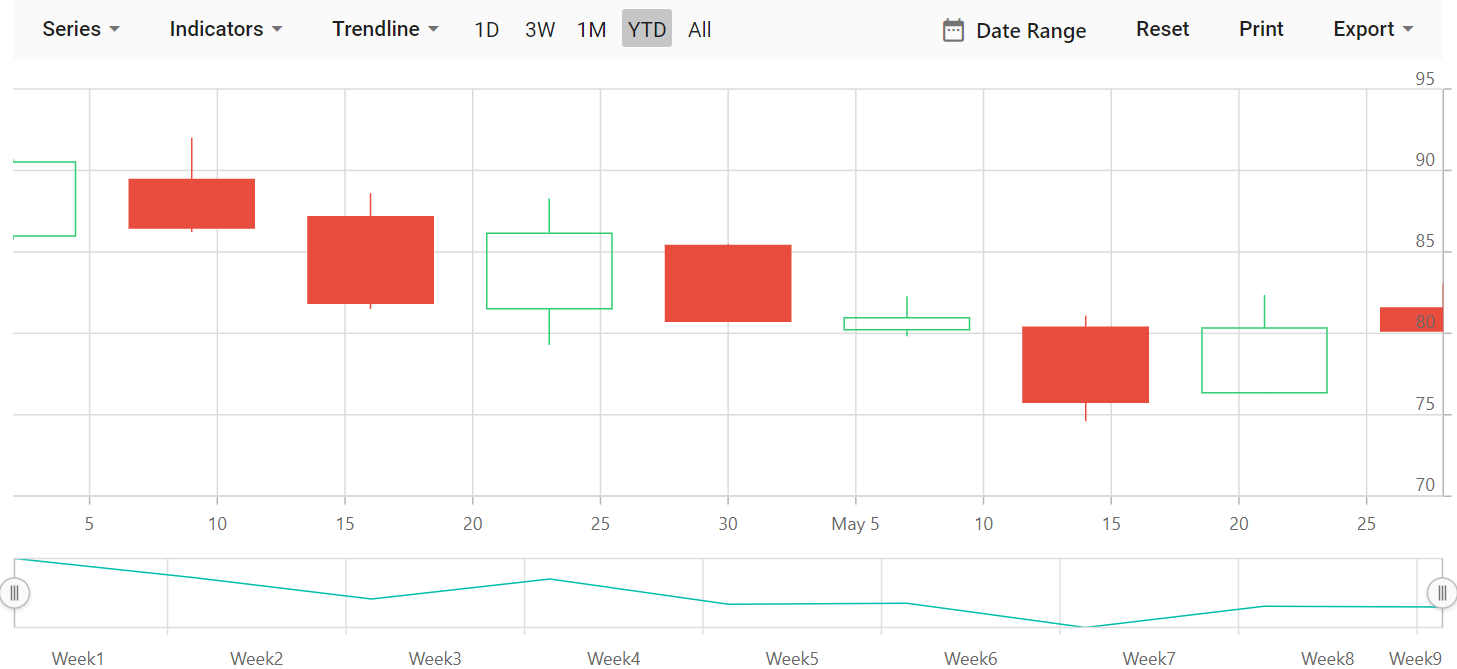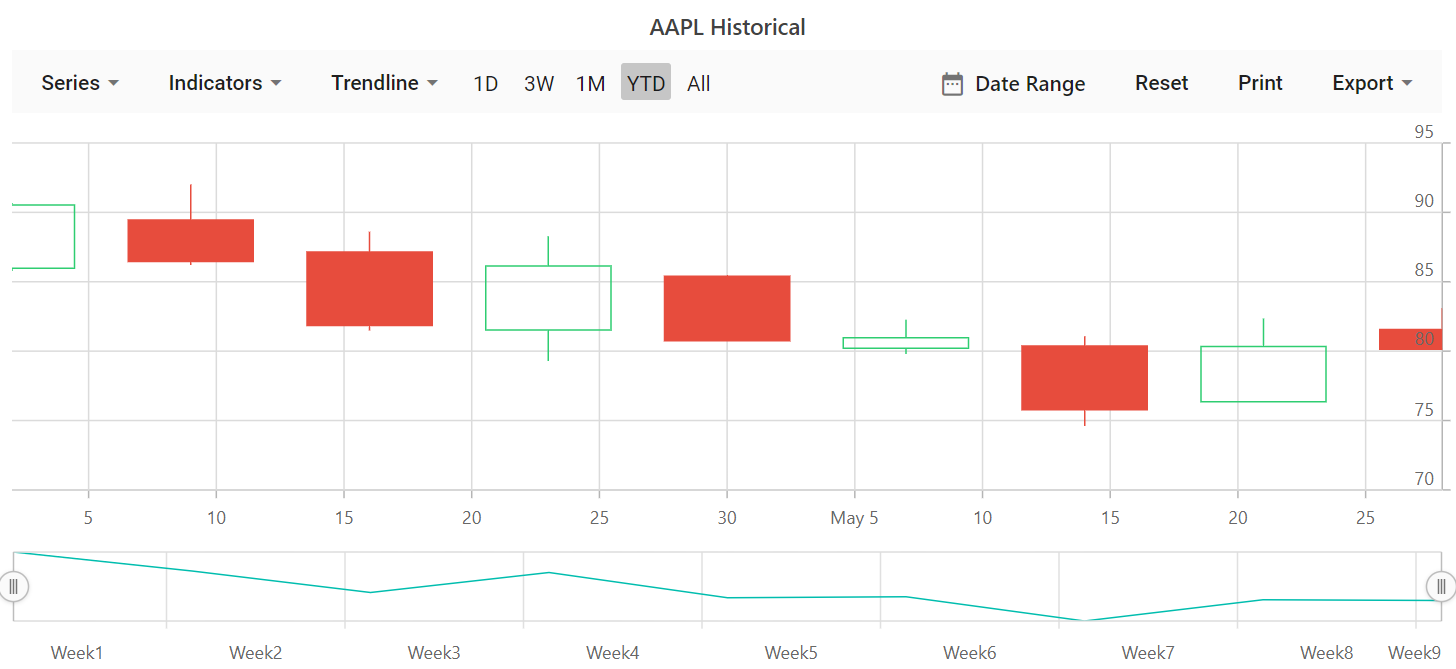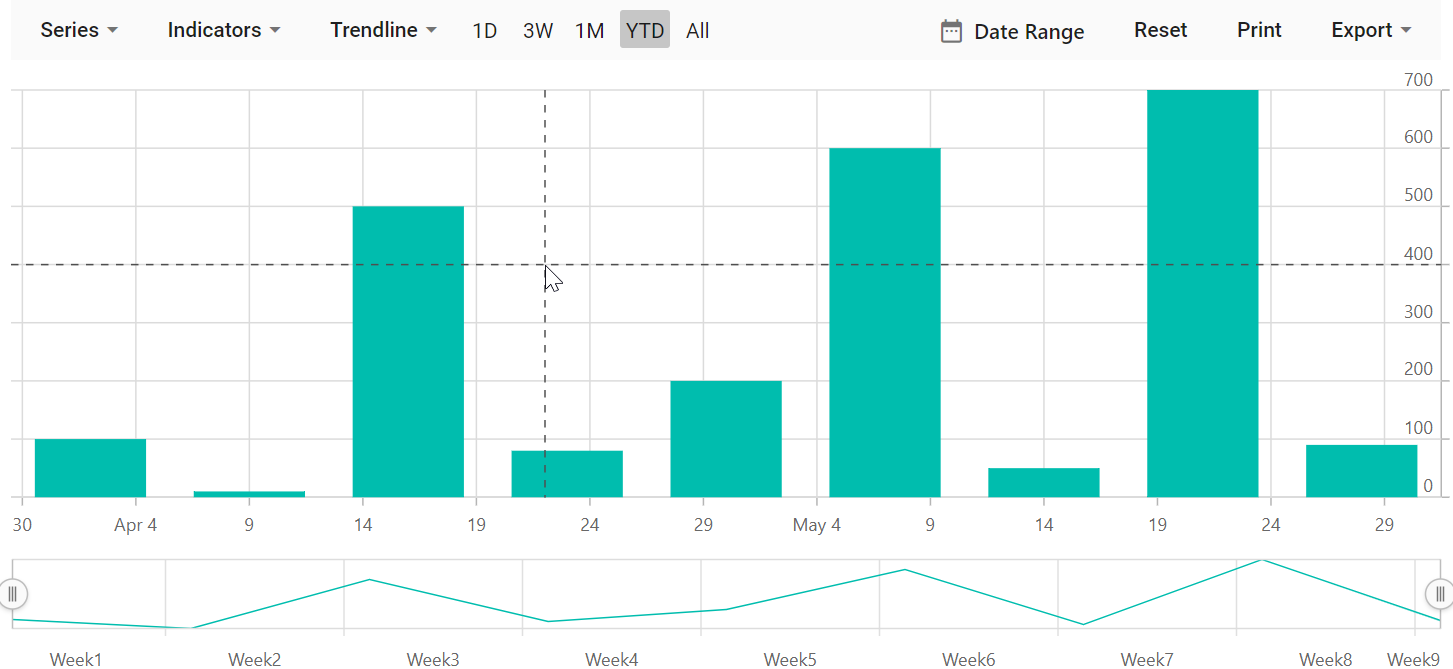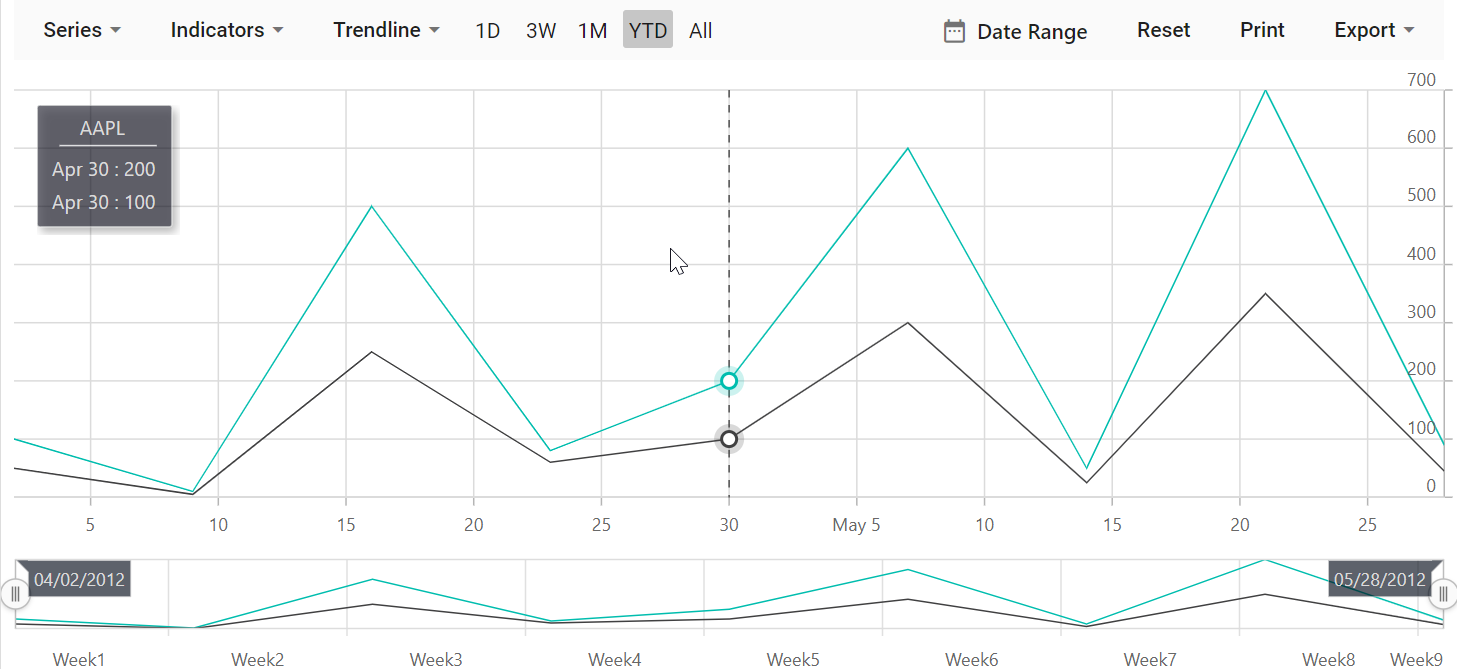# Getting Started with Blazor Stock Chart Component

8 Oct 202112 minutes to read

This section briefly explains how to include a Stock Chart component in the Blazor server-side application. Refer to Getting Started with Syncfusion Blazor for server-side in Visual Studio 2019 page for introduction and configuring common specifications.

To get start quickly with Blazor Stock Chart component, check on this video.

## Importing Syncfusion Blazor Stock Chart component in the application

1. Install Syncfusion.Blazor NuGet package to the application by using the NuGet Package Manager.

2. The client-side resources can be added through CDN or from NuGet package in the HEAD element of the ~/Pages/_Host.cshtml page.

`````` <head>
<!---CDN--->

For Internet Explorer 11, kindly refer the polyfills. Refer the documentation for more information.

`````` <head>
3. Now add the lodash script mandatorily to the HEAD element of the /Pages/Host.cshtml page, since it is used in the component.

`````` <head>
<script src="https://cdnjs.cloudflare.com/ajax/libs/lodash.js/4.17.20/lodash.min.js"></script>

## Adding component package to the application

Open the ~/_Imports.razor file and include the Syncfusion.Blazor.Charts namespace.

``@using Syncfusion.Blazor.Charts``

## Adding SyncfusionBlazor service in Startup.cs

Open the Startup.cs file and add services required by Syncfusion components using services.AddSyncfusionBlazor() method. Add this method in the ConfigureServices function as follows.

``````using Syncfusion.Blazor;

namespace BlazorApplication
{
public class Startup
{
....
....
public void ConfigureServices(IServiceCollection services)
{
....
....
}
}
}``````

To initialize the Stock Chart component, add the below code to Index.razor view page under ~/Pages folder. In a new application, if Index.razor page has any default content template, then those content can be completely removed and following code can be added.

``````@page "/"

<SfStockChart>

</SfStockChart>``````

## Populate Stock Chart with Data

To bind data for the Stock Chart component, assign a `IEnumerable` object to the DataSource property. It can also be provided as an instance of the DataManager.

``````@code{
public class StockChartData
{
public DateTime Date { get; set; }
public Double Open { get; set; }
public Double Low { get; set; }
public Double Close { get; set; }
public Double High { get; set; }
public Double Volume { get; set; }
}

public List<StockChartData> StockDetails = new List<StockChartData>
{
new StockChartData { Date = new DateTime(2012, 04, 02), Open = 85.9757, High = 90.6657, Low = 85.7685, Close = 90.5257, Volume = 660187068},
new StockChartData { Date = new DateTime(2012, 04, 09), Open = 89.4471, High = 92, Low = 86.2157, Close = 86.4614, Volume = 912634864},
new StockChartData { Date = new DateTime(2012, 04, 16), Open = 87.1514, High = 88.6071, Low = 81.4885, Close = 81.8543, Volume = 1221746066},
new StockChartData { Date = new DateTime(2012, 04, 23), Open = 81.5157, High = 88.2857, Low = 79.2857, Close = 86.1428, Volume = 965935749},
new StockChartData { Date = new DateTime(2012, 04, 30), Open = 85.4, High =  85.4857, Low = 80.7385, Close = 80.75, Volume = 615249365},
new StockChartData { Date = new DateTime(2012, 05, 07), Open = 80.2143, High = 82.2685, Low = 79.8185, Close = 80.9585, Volume = 541742692},
new StockChartData { Date = new DateTime(2012, 05, 14), Open = 80.3671, High = 81.0728, Low = 74.5971, Close = 75.7685, Volume = 708126233},
new StockChartData { Date = new DateTime(2012, 05, 21), Open = 76.3571, High = 82.3571, Low = 76.2928, Close = 80.3271, Volume = 682076215},
new StockChartData { Date = new DateTime(2012, 05, 28), Open = 81.5571, High = 83.0714, Low = 80.0743, Close = 80.1414, Volume = 480059584},
};
}``````

Now set the `StockDetails` to DataSource property. By default, Stock Chart will be rendered based on the provided `Date` and `High` fields value from datasource without any mapping.

``````<SfStockChart>
<StockChartSeriesCollection>
<StockChartSeries DataSource="@StockDetails" Type="ChartSeriesType.Candle" XName="Date" YName="Close" High="High" Low="Low" Open="Open" Close="Close" Volume="Volume"></StockChartSeries>
</StockChartSeriesCollection>
</SfStockChart>``````

On successful compilation of the application, the Syncfusion Blazor Stock Chart component will render in the web browser as shown below.A title using Title property can be added in the Stock Chart, to provide quick information to the user about the data plotted in the component.

``````@using Syncfusion.Blazor.Charts

<SfStockChart Title="AAPL Historical">
<StockChartSeriesCollection>
<StockChartSeries DataSource="@StockDetails" Type="ChartSeriesType.Candle" XName="Date" YName="Close" High="High" Low="Low" Open="Open" Close="Close" Volume="Volume"></StockChartSeries>
</StockChartSeriesCollection>
</SfStockChart>

@code {
public class ChartData
{
public DateTime Date { get; set; }
public Double Open { get; set; }
public Double Low { get; set; }
public Double Close { get; set; }
public Double High { get; set; }
public Double Volume { get; set; }
}

public List<ChartData> StockDetails = new List<ChartData>
{
new ChartData { Date = new DateTime(2012, 04, 02), Open= 85.9757, High = 90.6657,Low = 85.7685, Close = 90.5257,Volume = 660187068},
new ChartData { Date = new DateTime(2012, 04, 09), Open= 89.4471, High = 92,Low = 86.2157, Close = 86.4614,Volume = 912634864},
new ChartData { Date = new DateTime(2012, 04, 16), Open= 87.1514, High = 88.6071,Low = 81.4885, Close = 81.8543,Volume = 1221746066},
new ChartData { Date = new DateTime(2012, 04, 23), Open= 81.5157, High = 88.2857,Low = 79.2857, Close = 86.1428,Volume = 965935749},
new ChartData { Date = new DateTime(2012, 04, 30), Open= 85.4, High =  85.4857,Low = 80.7385, Close = 80.75,Volume = 615249365},
new ChartData { Date = new DateTime(2012, 05, 07), Open= 80.2143, High = 82.2685,Low = 79.8185, Close = 80.9585,Volume = 541742692},
new ChartData { Date = new DateTime(2012, 05, 14), Open= 80.3671, High = 81.0728,Low = 74.5971, Close = 75.7685,Volume = 708126233},
new ChartData { Date = new DateTime(2012, 05, 21), Open= 76.3571, High = 82.3571,Low = 76.2928, Close = 80.3271,Volume = 682076215},
new ChartData { Date = new DateTime(2012, 05, 28), Open= 81.5571, High = 83.0714,Low = 80.0743, Close = 80.1414,Volume = 480059584}
};
}``````The crosshair is a vertical and horizontal line on the view that shows the value of an axis when the mouse or touch is in a certain position. The crosshair lines can be enabled by using Enable property in the StockChartCrosshairSettings. Likewise tooltip label for an axis can be enabled by using Enable property in the StockChartTooltipSettings.

``````@using Syncfusion.Blazor.Charts

<SfStockChart>
<StockChartCrosshairSettings Enable="true"></StockChartCrosshairSettings>
<StockChartSeriesCollection>
<StockChartSeries DataSource="@StockDetails" Type="ChartSeriesType.Column" XName="Date" YName="Y"></StockChartSeries>
</StockChartSeriesCollection>
</SfStockChart>

@code {
public class ChartData
{
public DateTime Date { get; set; }
public Double Y { get; set; }
}

public List<ChartData> StockDetails = new List<ChartData>
{
new ChartData { Date = new DateTime(2012, 04, 02), Y= 100},
new ChartData { Date = new DateTime(2012, 04, 09), Y= 10 },
new ChartData { Date = new DateTime(2012, 04, 16), Y= 500},
new ChartData { Date = new DateTime(2012, 04, 23), Y= 80 },
new ChartData { Date = new DateTime(2012, 04, 30), Y= 200},
new ChartData { Date = new DateTime(2012, 05, 07), Y= 600},
new ChartData { Date = new DateTime(2012, 05, 14), Y= 50 },
new ChartData { Date = new DateTime(2012, 05, 21), Y= 700},
new ChartData { Date = new DateTime(2012, 05, 28), Y= 90 }
};
}``````The trackball is used to track a closest data point to the mouse or touch position. The trackball marker indicates the closest point and trackball tooltip displays the information about the point. It can be enabled by setting the Enable property in the StockChartCrosshairSettings to true and Shared property in the StockChartTooltipSettings to true in component.

``````@using Syncfusion.Blazor.Charts

<SfStockChart>
<StockChartCrosshairSettings Enable="true" LineType="LineType.Vertical"></StockChartCrosshairSettings>
<StockChartTooltipSettings Enable="true" Shared="true" Format="\${point.x} : \${point.y}" Header="AAPL"></StockChartTooltipSettings>
<StockChartSeriesCollection>
<StockChartSeries DataSource="@StockDetails" Type="ChartSeriesType.Line" XName="Date" YName="Y"></StockChartSeries>
<StockChartSeries DataSource="@StockDetails" Type="ChartSeriesType.Line" XName="Date" YName="Y1"></StockChartSeries>
</StockChartSeriesCollection>
</SfStockChart>

@code {
public class ChartData
{
public DateTime Date { get; set; }
public Double Y { get; set; }
public Double Y1 { get; set; }
}

public List<ChartData> StockDetails = new List<ChartData>
{
new ChartData { Date = new DateTime(2012, 04, 02), Y= 100, Y1= 50},
new ChartData { Date = new DateTime(2012, 04, 09), Y= 10 , Y1= 5},
new ChartData { Date = new DateTime(2012, 04, 16), Y= 500, Y1= 250},
new ChartData { Date = new DateTime(2012, 04, 23), Y= 80 , Y1= 60},
new ChartData { Date = new DateTime(2012, 04, 30), Y= 200, Y1= 100},
new ChartData { Date = new DateTime(2012, 05, 07), Y= 600, Y1= 300},
new ChartData { Date = new DateTime(2012, 05, 14), Y= 50 , Y1= 25},
new ChartData { Date = new DateTime(2012, 05, 21), Y= 700, Y1= 350},
new ChartData { Date = new DateTime(2012, 05, 28), Y= 90 , Y1= 45}
};
}``````The fully working sample can be found here.